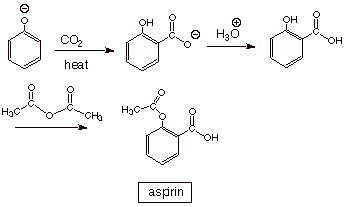# CH 610B

Second Exam

Spring,2002

Prof.N.L.Bauld

__________________________________________                                                                              Name (Last, First)

__________________________________________                                                                            Social Security Number

Page/Pts Earned

## Score

I.

22

2/11/

3/11/

II.

23

4/12/

5/11/

III.

19

6/9/

7/10/

IV.

15

8/7/

9/8

V.

23

10/10/

11/8/

12/5/

Totals

102

### I. Nuclear Magnetic Resonance (NMR)

A.The Basic Experiment

1. [3 pts] Assume a nucleus which has two spin states, designated as a  and  b Draw a diagram of the energies (E) of these two spin states on the Y axis vs. H (on the X axis) as a large, external magnetic field (H) is applied. Explain the qualitative effect of H upon each of these spin states.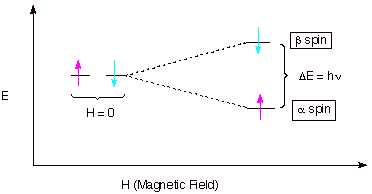q      The two spin states are of equal energy in the absence of the magnetic field.

q      When the field is applied, the alpha state, which is the state having the spin in the same direction as the applied field, decreases in energy, while the beta state, which has its spin opposed to the applied field increases in energy.

q      The energy difference increases as the magnitude of the field increases.

2. [2 pts] What is the requirement, expressed in terms of a mathematical equation, for such a nucleus to absorb radiation of frequency n? What happens to the spin state when this radiation is absorbed?

q      DE = hn.

q      The spin state which absorbs the radiation is converted into the other spin state.

3. [4 pts] Consider two extreme electronic forms of the hydrogen nucleus, i.e., the proton (H+) and the hydride ion (H-). Show on a plot of absorption (A) vs. magnetic field (H) qualitatively where these species would absorb relative to each other (high field or low field). Explain, with the assistance of another diagram, why a larger magnetic field is required to reach resonance for one of these species than the other. What is the name for this kind of magnetic effect?

q      In the presence of the large external magnetic field, the paired electrons around the nucleus of the hydride anion set up an induced magnetic field which is opposed to the direction of the applied field, thus making the net field at the nucleus less than the value of the applied field. Consequently,  a larger external applied field is needed to satisfy the resonance condition than in the case of a bare proton, which has no electrons around it.

q      This is called diamagnetic effect.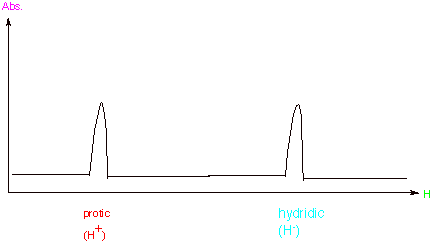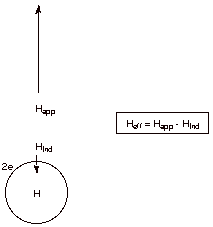B. Anisotropic Effects

1. [2 pts] Explain, with the assistance of a diagram, why the hydrogen nuclei of benzene or other aromatics absorb at very low fields. What is the approximate chemical shift of such a proton?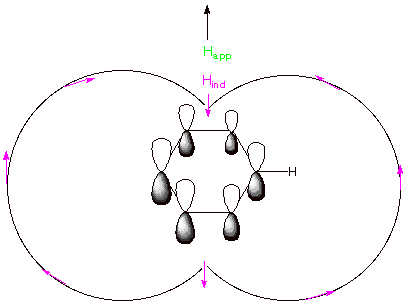2. [3 pts] What is the approximate chemical shift of an aldehydic proton? Explain this chemical shift qualitatively in terms of two distinct effects and give the names of the effects.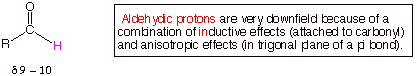q      The carbonyl carbon has a large partial positive charge, so that carbon is electronegative and draws electron density from the aldehydic hydrogen, making it more protonic. This is an electronegativity effect.

q      The carbonyl pi bond, like an alkene pi bond, has a diamagnetic effect which arises from the pi electrons, and shifts protons which are in the trigonal plane downfield.

q      The two effects combine to shift these protons to the very downfield region.

C. Simulated NMR Spectra

1. [6 pts] Provide a simulated NMR spectrum for the following molecule, mapping the spectrum from d = 0 to 10 or a smaller segment of the spectrum as needed. For each different set of protons (1) indicate which proton or group of protons in the given molecule give rise to this absorption (2) explain the chemical shifts of each set relative to  typical aliphatic methyl absorption at d = 0.9 in terms of an electronic effect,  and finally (3) explain the multiplicity of each set of protons, indicating what rule you are using to explain the multiplicities.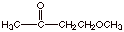q      The methyl and methylene groups attached to the carbonyl group are shifted downfield from aliphatic methyls by an electronegativity effect, since the attached carbonyl carbon is electron withdrawing.

q      The methoxy and methyleneoxy groups are shifted even more downfield because the oxygen atom attached to these carbons is highly electronegative.

q      The methyl attached to the carbonyl group is a single, because there is no vicinal hydrogen; the methoxy protons also are a singlet, since there are no vicinal protons on the neighboring oxygen; The two methylene groups are triplets as a result of the 2n+1 rule, i.e., each methylene group is split into a triplet because there are two vicinal protons (n=2).

q      It is important that the methyl and methylene protons attached to the carbonyl group are shown as absorbing at ca. d =2, but the sequence of these two is not important. Similarly, the methylene and methyl groups which are attached to oxygen should be shown as absorbing at ca. d = 3.5-4.0, but the sequence (up or down field is not crucial for this exam.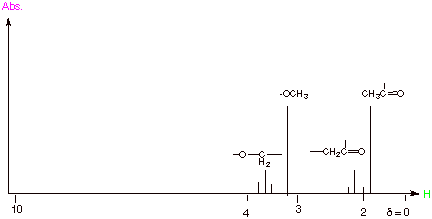D. Calibration of Chemical Shifts.

1. [2 pts] What molecule is used to calibrate the reference point d = 0? Why is this molecule particularly appropriate (at least two reasons)?

q      Tetramethylsilane (Me­­­4Si)

q      The TMS protons are somewhat hydridid because silicon is electropositive relative to carbon. These protons are therefore more upfield than most protons in strictly organic molecules.(This answer is required)

q      Also, TMS is a relatively unreactive and stable molecule, so it is compatible with most organic compounds.(Unreactive or stable is acceptable as the second answer.

# A. Thermodynamic Stability of Enols

1. [2 pts] Which is more stable, the keto form or the enol form shown below. Explain the basis for your conclusion in terms of bond strengths.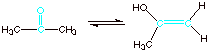q      The ketone form is more stable than the enol form, because the carbonyl double bond is much more thermodynamically stable than the alkene double bond present in the enol.

2. [4 pts] Provide a resonance theoretical treatment of the enol shown above and summarize as a dotted line/partial charge (DL/PC) structure. Is the enol double bond more or less reactive toward electrophiles (such as H+) than a simple alkene double bond? Explain in terms of a character revealed by your DL/PC.

The enol double bond is much more reactive toward electrophiles because it has a substantial partial negative charge accumulation, i.e., it has carbanion character.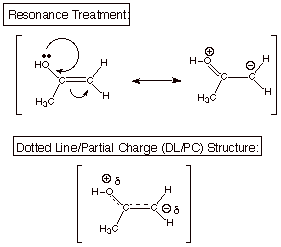B. Mechanism of Acid Catalyzed Enolization

1. [3 pts] Write the detailed mechanism for the acid catalyzed enolization of acetone (the ketone form shown in the above question) in aqueous solution containing strong acid (hydronium ion).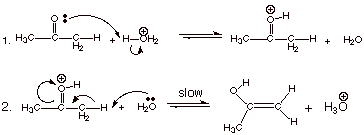C. Mechanism of Base Catalyzed Enolization

1. [3 pts] Write the detailed mechanism for the base catalyzed enolization of acetone.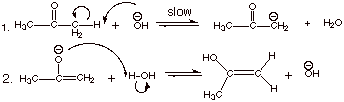**Note that either canonical form (or both) of the enolate can be written here.

D. Acidity of Carbonyl Compounds

1. [2 pts] What is the approximate pKa value of a simple ketone like acetone. Is acetone more or less acidic than water? What is the pKa of water?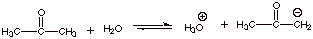q      The approximate pKa is 19 (accept 18-20)

q      Acetone is therefore less acidic than water.

q      Water has a pKa of about 16 (15.7).

2. [2 pts] Provide a resonance theoretical treatment of the acetone enolate, summarize as a dotted line/partial charge structure, and characterize this structural model.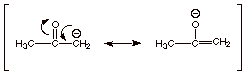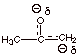q      Oxyanion (alkoxide) character

q      Carbanion character

3. [3 pts] Referring to your resonance structures for the enolate, indicate a factor which favors each of the canonical structures (one factor favoring the structure with negative charge on carbon and one favoring the structure with negative charge on oxygen). What is the relevance of these counteracting factors to the magnitude of the resonance stabilization?

q      The structure with oxyanion character tends to be favored because oxyanion character is more favorable than carbanion character.

q      Thes structure with carbanion character tends to be favored because it  has a carbonyl double bond, which is more stable than the alkene double bond present in the oxyanion structure.

q      These factors are relevant in that they tend to oppose and cancel one another, bringing the two structures very close together in energy, a circumstance which maximizes the resonance stabilization of the enolate.

4. [4 pts] Consider the acid/base reaction below and use a qualitative criterion to predict whether the equilibrium goes to the product or the reactant side. State the criterion you are using. Then, state and apply a quantitative criterion to obtain the actual equilibrium constant for this acid/base reaction.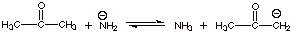q      Qualitative Criterion: “The stronger acid is converted to the weaker acid.” The stronger acid is acetone, which has a pKa of ca. 19, while the weaker acid is NH­3 , which has a pKa of 38. Thereforfe the reaction goes to the right as written.

q      Quantitative Criterion: K = K­a (Reactant Acid)/Ka (Product Acid)

K = 10-19/10-38 = 1019

Therefore the reaction goes far to the right, specifically with the K value shown.

# A.Mechanism of the Aldol Addition Reaction

1.    [4 pts] Write the detailed mechanism for the base catalyzed aldol addition reaction of propanal. Provide the IUPAC name and the structure of this product.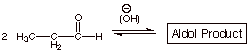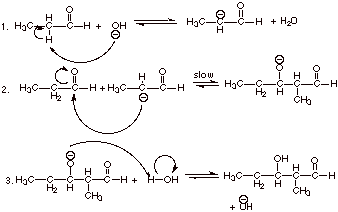q      The IUPAC name of the aldol product is 3-hydroxy-2-methylpentanal.

2. [3 pts] Provide the structures of 2 aldehydes which do not undergo the aldol reaction and explain why.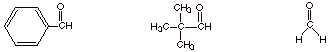q      These three aldehydes (only two are required) exemplify aldehydes which do not have an alpha hydrogen and therefore cannot form an enolate, which is required in the aldol reaction. (Note that the last one doesn’t even have an alpha carbon).

B.Crossed Aldol Reactions.

1. [2 pts] Explain why the crossed aldol reaction between ethanal and propanal is not synthetically useful.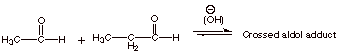q      There are two different enolates (one from each aldehyde) and two different carbonyl components (aldehydes). Therefore, there are four different aldol products, all of which would be formed competitively.

2. [3 pts] Draw the structure of the product of the following successful crossed aldol reaction and explain why this reaction works efficiently. Which reactant should be added last and in a dropwise manner? Explain why this is important in obtaining good yields of the crossed aldol adduct.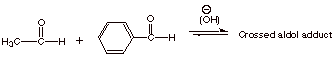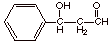q      Only acetaldehyde (ethanal) can form an enolate, so there is only one enolate which can be formed. The cross product must be that of this enolate with benzaldehyde as the carbonyl component, as shown.

q      Acetaldehyde should be added last, so that the concentration of benzaldehyde is always in excess. This is so that the enolate of acetaldehyde will prefer to add to benzaldehyde (present in excess) over addition to acetaldehyde, which would give the regular aldol product of acetaldehyde.

C.Mechanism of the Aldol Condensation Reaction

1. [2 pts] Write the detailed mechanism for the second stage of the aldol condensation reaction, given in the equation below: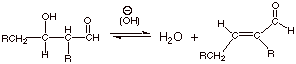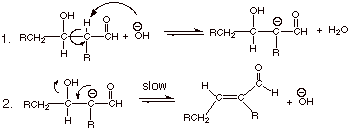2. [1 pts] What is unusual about the leaving group in this reaction?

q      The leaving group is hydroxide anion, which is not a really good leaving group.

3. [1 pts] Is this elimination mechanism of the E2 type? Explain why or why not.

q      No, this mechanism is not of the E2 type. The elimination is not concerted, like an E2 mechanism would be. Instead, it occurs in a stepwise manner, via the intermediate enolate.

D. Intramolecular Aldol Reaction

1. [3 pts] Draw the structure of the enolate which would be formed from the following difunctional aldehyde in aqueous base, and indicate by electron flow arrows how it would react intramolecularly (within the same molecule). Draw the structure of the aldol addition product. What is unique about the structures of the products of the intramolecular aldol reaction.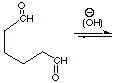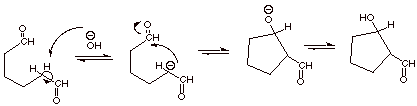## IV. Claisen Ester Condensation; Acetoacetic and Malonic Ester Syntheses

A.Mechanism of the Claisen Ester Condensation

1. [3 pts] Write the detailed mechanism for the Claisen ester condensation of ethyl acetate. You may use EtO- and EtOH to abbreviate ethoxide and ethanol. Similarly, you may use Et in the ester to abbreviate the ethyl group.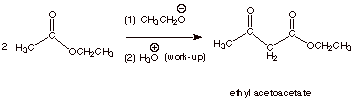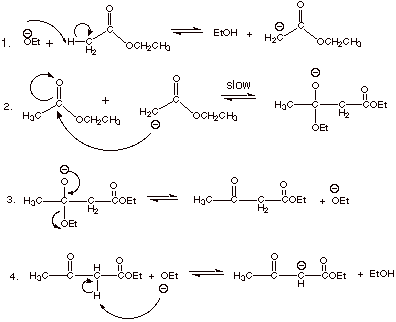2. [2 pts] Is the conversion as written above, i.e., the conversion of two molecules of ethyl acetate to one of ethyl acetoacetate (plus one molecule of ethanol) energetically favorable? To put it another way, is the result of the first three steps of your mechanism (if written correctly) a favorable conversion? Why or why not? Why does the Claisen condensation succeed?

q      The conversion of an ester functionality to a ketone functionality is unfavorable energetically, because of the resonance stabilization of the ester function. Therefore the result of the first three steps is not favorable for the reaction.

q      The Claisen condensation succeeds because the last step, a highly exergonic acid/base reaction, provides the thermodynamic driving force.

3. [2 pts] Provide a resonance theoretical treatment of the conjugate base of  ethyl acetoacetate, and summarize it as a dotted line/partial charge (DL/PC) structure.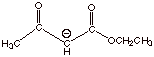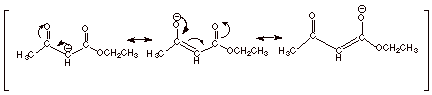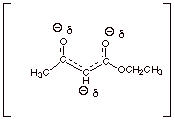4. [2 pts] What is the pKa of ethyl acetoacetate? Of Ethyl acetate? Explain the difference.

q      The pKa of ethyl acetoacetate (acetoacetic ester)is ca. 10.

q      The pKa of ethyl acetate is ca. 21.

q      The enolate of acetoacetic ester is more highly resonance stabilized than that of ethyl acetate, because the former has three resonance structures, while the latter has only has two. (To put it differently, the negative charge of the enolate of ethyl acetoacetate is delocalized over a more extended conjugated system than that of ethyl acetate.

5. [1 pts] Provide an estimate of the pKa of the ester shown below and explain the basis for your prediction.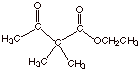q      The pKa is about 19, that is about that of an ordinary ketone which has alpha hydrogens.

q      The carbon atom which is between the two carbonyl groups has no alpha type hydrogens attached, so it does not have the extra acidity of beta dicarbonyl compounds.

B. Acetoacetic Ester Syntheses

1. [4 pts] Sketch a synthesis of the following ketone using ethyl acetoacetate and any alkyl halide having 4 carbons or less.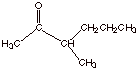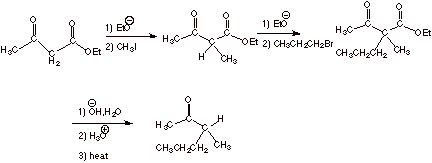#### . Aromaticity

A.Aromaticity of Benzene

1. One canonical structure for benzene is illustrated below. What is the name given to this structure of benzene?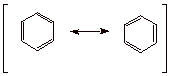Kekule structure

2. In the space provided above in question V.A.1, complete the usual resonance theoretical treatment of benzene , and indicate in the space below this what are the energetic and geometric results of this resonance stabilization. Be as quantitative and specific as you can about both the energetic and geometric consequences of benzene’s resonance stabilization.

q      Benzene is highly resonance stabilized, i.e., its energy is lowered by at least 36 kcal/mol compared to cyclohexatriene.

q      Instead of having alternate double and single bonds, benzene is a regular hexagon, with all bonds being equivalent and 1.39 A long.

3. Explain in terms of the molecular orbital picture of the pi electronic system of benzene provided below, why benzene is aromatic (strongly resonance stabilized). What is the significance of the dashed line? What are the MO’s which fall below the dashed line called? Those which appear above the dashed line?q      The dashed line represents the non-bonding energy leve,i.e., the energy of an electron in an atomic orbital (2p) on carbon.

q      Orbitals which are more stable (below the non-bonded line) are called bonding molecular orbitals (BMO’s).

q      Orbitals which are less stable (above the NB line) are called antibonding molecular orbitals (ABMO’s).

q      Benzene is so highly resonance stabilized because there are three strongly bonding MO’s and the six electrons in the pi system of benzene fill all three of the BMO’s and none of the ABMO’s. [Give +1 extra credit if it is mentioned that the most bonding MO in cyclic systems is especially low in energy]

4. Using the mnemonic device we developed in class (called the Frost-Musulin mnemonic) provide a similar diagram of the MO’s of the pi electron system of cyclobutadiene. Is this system aromatic? Explain why or why not. (Do not use the Huckel rule to explain this.)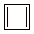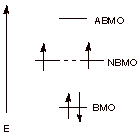q      The MO’s follow the pattern of a square, with one vertex down. This means there is only one BMO. The next two are at the non-bonding level.

q      The four electrons of the two double bonds (formally) of cyclobutadiene cannot all go into bonding orbitals. Two of them go into non-bonding orbitals (NBMO’s)which provide no molecular bonding.

q      Consequently, cyclobutadiene is antiaromatic (or non-aromatic).

q      [1 point extra credit if it is mentioned that the electrons go into different NBMO’s with their spins unpaired, according to the Hund Rule.

5. The molecule shown below is called pyridine. Is pyridine aromatic or not? Explain why or why not, including in your explanation the unshared pair on nitrogen. Also, draw an orbital picture of the pi system of pyridine, showing the overlap of the 2p AO’s and also the orbital occupied by the unshared pair on nitrogen.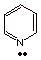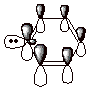q      Pyridine is aromatic. It has a six orbital, 6 electron pi system just like benzene.

The unshared pair is in an sp2 AO on nitrogen, which is perpendicular to the 2pz AO’s which form the aromatic system, and are not a part of the pi system.

B. Systems Other Than Benzene.

1. Classify the conjugated pi electron systems shown below as aromatic or antiaromatic (or, if you prefer, non-aromatic), explaining your answer and showing how you are counting electrons.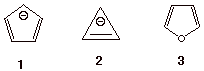q      The cyclopentadienyl anion (1) is aromatic. It has a pi system containing 6 electrons, which conforms to the requirements of the Huckel 4n+2 rule, with n=1. .The electrons are counted as follows: Two electrons for each of the double bonds (total of 4), and two electrons for the carbanion center.

q      The cyclopropenyl anion (2) is antiaromatic, since it has 4 electrons (4n). These come from one double bond and one carbanion center.

q      The third molecule, furan, is aromatic. The divalent oxygen has two unshared pairs. One of them is in the trigonal plane and not a part of the pi system, but the other is in the pi system. These two electrons make the electron count 6.

C. Phenol

1.What is the approximate pKa of phenol? Compare this to the pKa of an ordinary alcohol. By what factor is phenols acidity increased or decreased? Which is it, increased or decreased?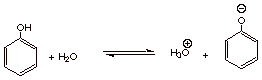q      pKa of phenol is ca. 10.

q      pKa  of typical alcohols is ca. 16.

q      The acidity of phenols is greater by 106 (one million times).

2.    Using resonance theory, explain the sharp difference in acidities of ordinary alcohols and phenols.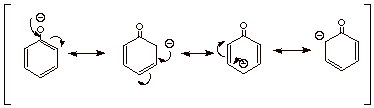q      The phenoxide anion, the conjugate base of phenol, is highly resonance stabilized, with the negative charge being delocalized onto the ortho and para positions of the benzene rin, as shown in the four canonical structures above.

q      This makes the phenoxide anion much more thermodynamically stable than an alkoxide anion, the conjugate base of an ordinary alcohol. Consequently, the acid dissociation equilibrium for phenol lies much farther to the right, producing much more hydronium ion.

3. What base can be used to separate phenols from carboxylic acids and ordinary alcohols by means of acid/base extraction techniques? Explain, in terms of the qualitative theory which allows one to predict when an acid/base reaction will go to completion, why this method works.

q      Bicarbonate is basic enough to neutralize a carboxylic acid, but not enough to neutralize phenol. The carboxylate anion is thus extracted into the aqueous phase as the sodium salt, while the phenol remains soluble in the organic phase.

q      This works because the stronger acid in an acid/base reaction goes to the weaker acid (see below). Carbonic acid, the conjugate acid of bicarbonate anion, is a stronger acid than phenol, so the equilibrium favors the weaker acid, phenol. On the other hand, carboxylic acids (pKa ca. 5) are stronger acids than carbonic acid (pKa ca. 7), so carboxylic acids are converted to carboxylate anions and carbonic acid.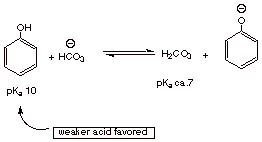4. Sketch an efficient synthesis of aspirin.